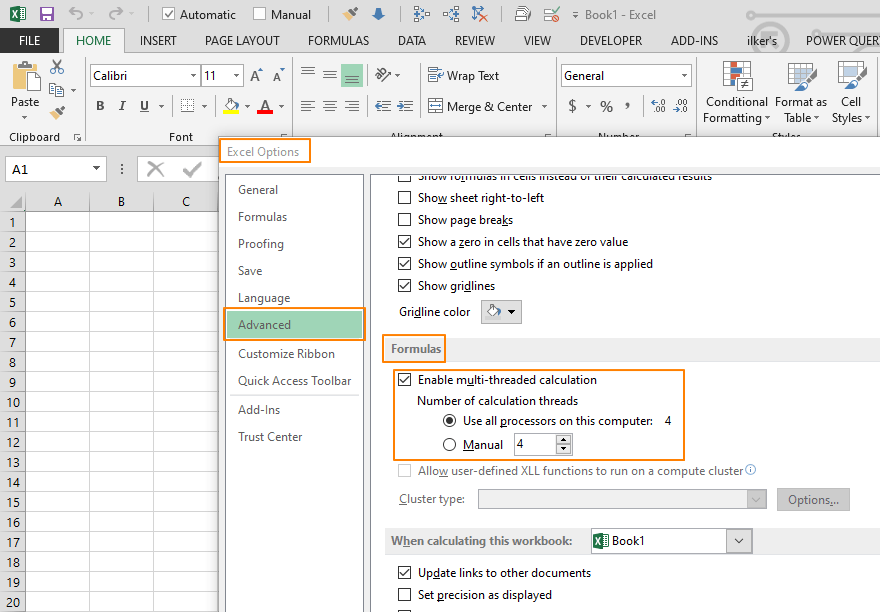Excel is a fairly an efficient software given that it can perform complex calculations. However, depending on your system, it can also get pretty slow when running complicated calculations. How formulas are used, and size of the workbook are typically the culprit if your workbook takes minutes to calculate. In this guide we're going to show you how to make Excel calculate faster.

## Try using "faster formulas"

Not all formulas are created equal, and some of them simply run slower than the others. Some functions can do what another function can much faster. Of course, the performance gain will be different from one scenario to the other - we will offer some advise on which functions could prove faster than others.

Typically, complexity and calculation times are inversely proportional. For example, using the SUMIFS function instead of SUMPRODUCT or SUM functions in an array formula can yield better results. Similarly, SUMIF function can be significantly faster than SUMIFS. However, we do not suggest using SUMIF unless necessary.

Another cool trick here is using two approximate VLOOKUPs instead of a single exact VLOOKUP. For more tips about lookup functions, please see How to speed up lookup formulas.

Overall, prefer using simpler formulas and helper columns instead of one big formula!

A final tip: if you are using a formula like =IF(A1>0,A1,0), you can use something like =MAX(A1,0) for better performance.

## Avoid volatile formulas

Excel is smart, and calculates only dependent cells when you make changes to a cell. However, some of functions are calculated doesn't follow the same logic - They are calculated every time. Thus, they have an impact on calculation times.

If your workbook suffers from substantial performance losses, we advise avoiding them as much as you can. Here is a list of the functions you might want to avoid:

• NOW()
• TODAY()
• RAND()
• RANDBETWEEN()
• OFFSET()
• INDIRECT()
• INFO() (depending on its arguments)
• CELL() (depending on its arguments)

You can learn more about volatile functions in our article: Handle With Care: Volatile Functions in Excel

## Avoid large ranges

A larger range means more cells to be evaluated, and more cells means more places to process. This element also has an impact on calculation times, and it might be a good idea to check the ranges used in formulas. Although selecting a whole column is easier than selecting a range, prefer using ranges for best results and avoid empty cells at the end of your work area.

## Replace formulas with values

This might sound obvious, but it's always good to double-check the ranges used in formulas. In a complex workbook some range may contain formulated data not updated by any input. Making them static removes them calculation chain of Excel. This may reduce file size and speed opening and saving times up.

## Avoid conditional formatting

Conditional formatting can substantially enhance the user experience. So, you should use formatting features. On the other hand, formatting is not needed for the calculations. Try to remove the conditional formatting rules for performance gain.

## Decrease the number of worksheets

Excel calculates a workbook faster if data and formulas reside in the same worksheet. Try using fewer worksheets in your workbook!